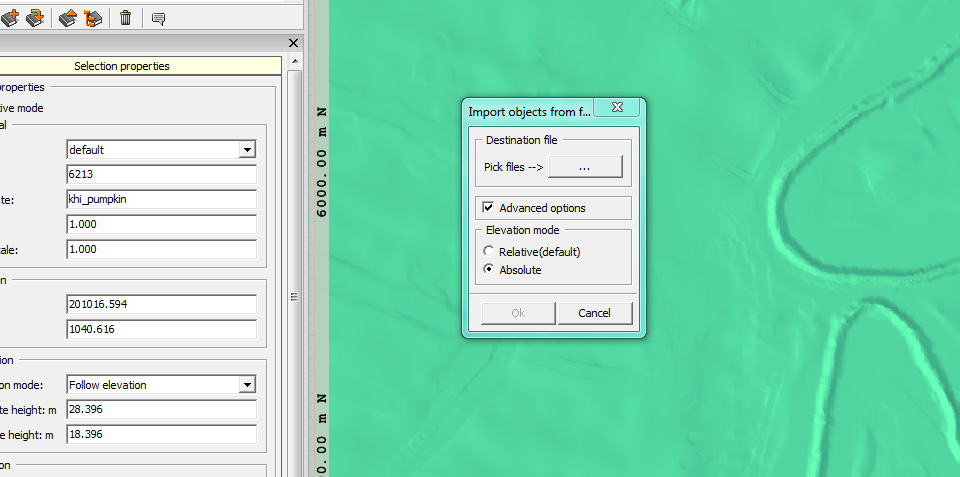# Exporting from Arma 3 to Terrain Builder

This is an attempt to have a script that can accurately export from Arma 3 (either inside a mission or from Eden) to a Terrain Builder object list (.txt) file. If it survives a bit more testing, this page will be updated with some more details.

In the meantime, to test it out, you can download the following patch .pbo to fix the Export to Terrain Builder option in the 3DEN menu. It should faithfully export all of the rotations and heights:

If you've installed it correctly, all of the columns of the export will have numbers with six decimal places:

```"axis";204080.484375;2083.952881;0.000000;75.000000;0.000000;1.000000;207.011108
```

If it is incorrectly installed, and you are still using the original 3DEN export, your result will look more like this:

```"axis";201328.015625;2544.666016;0;75;0;1;207.011108;
```

When importing in to Terrain Builder, it is important to remember to import with absolute heights.

Please let me (ianbanks in the Arma 3 Discord, or on the above e-mail address) know if you have any issues with it.

The script used in the patch is:

```khi_fnc_matrixVectorMultiply = {
params ["_matrix", "_v"];

_matrix params ["_x", "_y", "_z"];

[
_x vectorDotProduct _v,
_y vectorDotProduct _v,
_z vectorDotProduct _v
]
};

khi_fnc_getTerrainBuilderMatrix = {
params ["_x", "_y", "_z"];

[
[cos _y * cos _z - sin _x * sin _y * sin _z, cos _z * sin _x * sin _y + cos _y * sin _z, cos _x * sin _y],
[-cos _x * sin _z, cos _x * cos _z, -sin _x],
[-cos _z * sin _y - cos _y * sin _x * sin _z, cos _y * cos _z * sin _x - sin _y * sin _z, cos _x * cos _y]
]
};

khi_fnc_getTerrainBuilderAngles = {
private _direction = vectorDir _this;
private _up = vectorUp _this;
private _aside = _direction vectorCrossProduct _up;

private ["_xRot", "_yRot", "_zRot"];

if (abs (_up select 1) < 0.999999) then {
_xRot = -asin (_up select 1);

private _signCosX = if (cos _xRot < 0) then { -1 } else { 1 };

_yRot = ((_up select 0) * _signCosX) atan2 ((_up select 2) * _signCosX);
_zRot = (-(_aside select 1) * _signCosX) atan2 ((_direction select 1) * _signCosX);
} else {
_zRot = 0;

if (_up select 1 < 0) then {
_xRot = 90;
_yRot = (_direction select 0) atan2 (_direction select 2);
} else {
_xRot = -90;
_yRot = (-(_direction select 0)) atan2 (-(_direction select 2));
};
};

[_xRot, _yRot, _zRot]
};

khi_terrainBuilderMapFrameOffset = [200000, 0, 0];

khi_fnc_getTerrainBuilderObjectName = {
getModelInfo _this params ["_name"];

if (count _name < 4 ||
{ _name select [count _name - 4, 4] != ".p3d" }) exitWith { _name };

_name select [0, count _name - 4]
};

khi_fnc_getTerrainBuilderLine = {
params ["_object", ["_scale", 1], ["_name", ""]];

_object call khi_fnc_getTerrainBuilderAngles params ["_xRot", "_yRot", "_zRot"];

private _centre = getPosWorld _object;

private _terrainBuilderMatrix = [_xRot, _yRot, _zRot] call khi_fnc_getTerrainBuilderMatrix;
private _terrainBuilderGround = [_terrainBuilderMatrix, boundingCenter _object] call khi_fnc_matrixVectorMultiply;

private _terrainBuilderScaledGround = _terrainBuilderGround vectorMultiply _scale;

private _terrainBuilderPosition = _centre vectorDiff [0, 0, _terrainBuilderScaledGround select 2];

params ["_xPos", "_yPos", "_zPos"];

private _values = [_xPos, _yPos, _zRot, _xRot, _yRot, _scale, _zPos] apply { _x toFixed 6 };

if (_name == "") then { _name = _object call khi_fnc_getTerrainBuilderObjectName; };

private _line = [str _name] + _values joinString ";";

_line
};

khi_fnc_getTerrainBuilderLines = {
private _lines = [];

{
_lines pushBack ([_x] call khi_fnc_getTerrainBuilderLine);
}
forEach _this;

_lines joinString toString [13, 10]
};
```

To use the script manually in Eden, first execute the above snippet in the Eden debugger, and then run:

```copyToClipboard (all3DENEntities select 0 call khi_fnc_getTerrainBuilderLines);
```

If all goes well, your clipboard will contain the object list. Note that when importing to Terrain Builder, absolute heights must be used, and also that if you call khi_fnc_getTerrainBuilderLine directly with an object scale, the scaling will be from the object centre rather than the ground contact point.